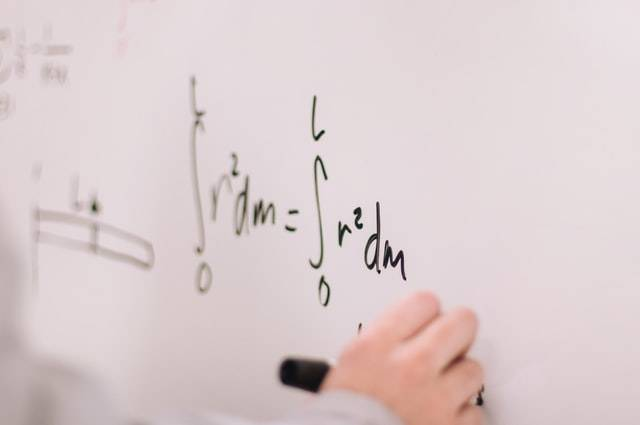# How do Math Worksheets Help in Learning Algebraic Formulas?

0
193Algebra is the broadest topic that falls under mathematics. It covers everything right from the operations performed on numbers to the formation of complicated mathematical equations. We require algebraic formulas that act as a key to solving any math question. There are several formulas that have to be on a child’s fingertips when he appears for an exam. This can prove to be a daunting task. This article will see how math worksheets help strengthen a kid’s concepts in algebra.

The questions provided in the worksheets are presented in a well-organized manner. More straightforward questions and easier sections make up the starting problems of these worksheets. As a child applies the formulas to these sums and develops his confidence, he can move on to tougher sections that consist of more complicated problems. Math worksheets are also equipped with answer keys that help kids to figure out where they make mistakes while attempting these problems. It helps them in clearing doubts before proceeding further.

## 1. Understanding a Math Formula

Before a kid can move to solve questions based on a particular formula, he needs to understand how it was formed. Thus, he needs to go through the derivation of the procedure before applying it practically. Once he gains an in-depth knowledge of the steps that go into producing an algebraic formula, he creates a strong foundation of the basics. During an exam, if a child forgets the final representation of a specific formula, he can easily derive it and come to a conclusion. Thus, he does not lose marks because he failed to memorize that formula.

See also  Should You Declare A College Minor?

## 2. Practice Questions

It is very important to understand the concept behind a formula; however, it is equally essential for students to memorize the same. If a kid gets stuck during an exam, he can derive the formula because he has crystal clear concepts; however, this can prove to be time-consuming. Timed examinations usually do not provide extra minutes for figuring out how to reach a formula. The only way to remember such a large number of formulas is by solving the questions given in the math worksheets for 4th grade. As kids attempt the sums and start applying the procedures they were taught, it gets stored in their muscle memory. At one point, they will become comfortable enough with using these that they will not have to think about which formula to apply in what question. Thus, practice makes a child perfect, and these worksheets are the ideal place to get a wide-ranging variety of sums.

## 3. Well-structured

The questions provided in the worksheets are presented in a well-organized manner. More straightforward questions and easier sections make up the starting problems of these worksheets. As a child applies the formulas to these sums and develops his confidence, he can move on to tougher sections that consist of more complicated problems. Math worksheets are also equipped with answer keys that help kids to figure out where they make mistakes while attempting these problems. It helps them in clearing doubts before proceeding further.

## 4. Interactive and Visually Simulating

As most worksheets are online, the questions come with relevant and fun graphics to give kids a better idea of how to start solving a problem. It improves their visualization skills that can be transferred to any topic or subject. As the worksheets are interactive, kids can come up with their own creative ways of solving questions.

See also  Improve Your Writing Skills with 5 Foolproof Paraphrasing Techniques

## Conclusion about Math Worksheets

Worksheets are very important, as we can see from the points listed above. However, the issue lies in finding good worksheets to help a child supplement his learning rather than confuse him. Cuemath is an excellent online educational platform that provides well-curated resources to study and gives its students access to other assets such as math puzzles, games, apps, etc. The certified math tutors focus on concept building, and good grades follow as a consequence. Hopefully, this article gives you an insight into how to learn algebraic formulas, and I wish you all the best!

Đánh giá bài viết